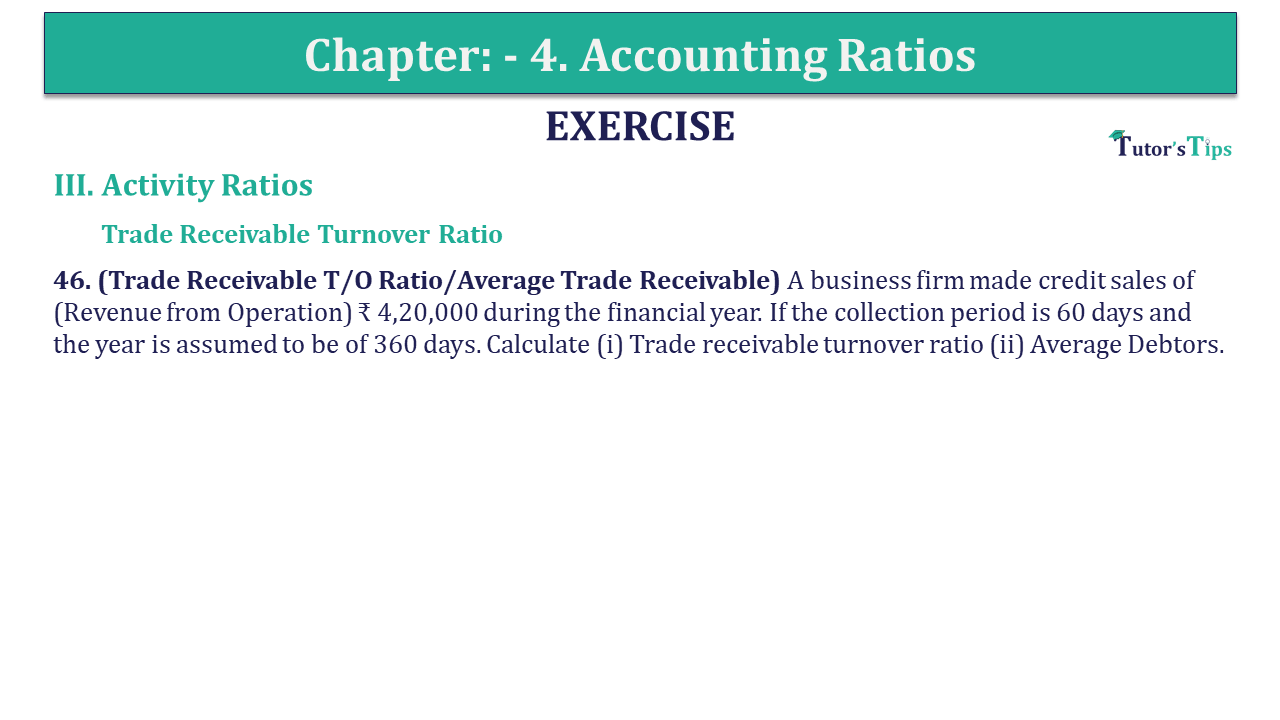# Question 46 Chapter 4 of +2-B – USHA Publication 12 ClassQ-46- CH-4 Book 2 - Usha Pub. +2 Book 2020 - Solution

Question 46 Chapter 4 of +2-B

46. (Trade Receivable T/O Ratio/Average Trade Receivable) A business firm made credit sales of (Revenue from Operation) ₹ 4,20,000 during the financial year. If the collection period is 60 days and the year is assumed to be of 360 days. Calculate (i) Trade receivable turnover ratio (ii) Average Debtors.

## The solution of Question 46 Chapter 4 of +2-B: –

 Trade Receivable Turnover Ratio = Number of days Average Collection Period Trade Receivable Turnover Ratio = 360 60 = 6 times
 Average collection period = Net Credit Sales Average Trade Receivable = ₹ 4,20,000 Average Trade Receivable Average Trade Receivable = ₹ 4,20,000 6 = ₹ 70,000

Also, Check out the solved question of previous Chapters: –